##### Actions

Introduced in 1964 by J.E. Broadwell, this model is the classic example of a discrete velocity gas. A discrete velocity model consists of a collection of gas molecules with velocities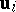belonging to some finite setof discrete velocity vectors in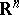. The molecules collide with each other, obeying specified sets of transformation rules which must satisfy basic conservation laws. The models generate systems of highly coupled semi-linear partial differential equations which approximate the Boltzmann equation, and are particularly useful for studying problems in rarefied gas dynamics, such as Couette flow, Rayleigh flow and shock structure, especially at high Mach number (cf. also Gas dynamics, numerical methods of; Gas flow theory; Gas dynamics, equations of).

In the Broadwell model, each identical molecule of massis allowed to move in space with one of the six unit velocity vectors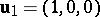,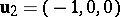,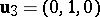,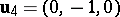,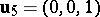,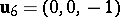. Gas particles are allowed to collide only in pairs, a realistic assumption for gases which are not too dense, and the collision must obey the usual conservation laws for mass, momentum and kinetic energy. Let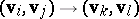denote a collision of particles with initial velocities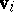andand final velocities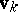and. Conservation of momentum dictates that the only possible collisions are then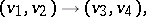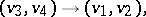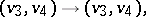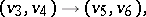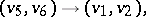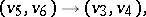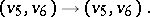In each of the above collisions, both mass and kinetic energy are also preserved.

Lettingdenote the number density of molecules with velocity, the Boltzmann equation can be written as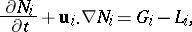whereand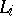are the rates of gain and loss in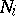as a result of collisions. Assuming spherical symmetry and collisional cross section, one has, for example,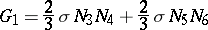andsince one-third of the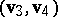andcollisions yield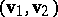pairs.